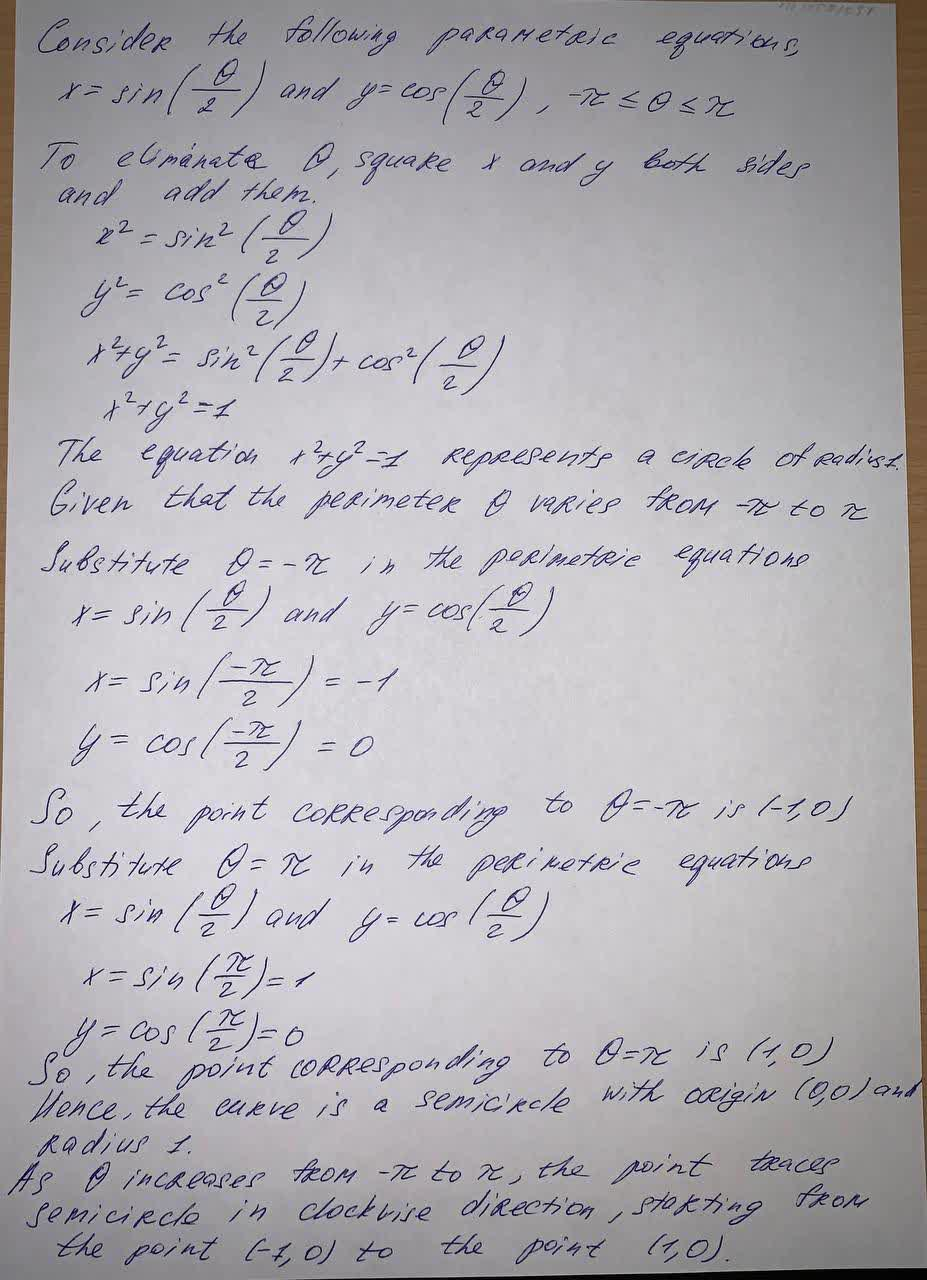Question# x =\sin \left(\frac{\theta}{2}\right), y = \cos \left(\frac{\theta}{2}\right), -\pi \leq \theta \leq \pi (a) Eliminate the parameter to find a Cartesian equation of the curve. and how does thecurve look

Integrals
ANSWERED$$x =\sin \left(\frac{\theta}{2}\right), y = \cos \left(\frac{\theta}{2}\right), -\pi \leq \theta \leq \pi$$ (a) Eliminate the parameter to find a Cartesian equation of the curve. and how does thecurve look2021-05-092021-09-30

Consider the following parametric equations,

$$x=\sin(\frac{\theta}{2})$$ and $$y=\cos(\frac{\theta}{2}),\ -\pi\leq\theta\leq\pi$$

To eliminate $$\theta$$, square x and y both sides and add them

$$x^2=\sin^2(\frac{\theta}{2})$$

$$y^2=\cos^2(\frac{\theta}{2})$$

$$x^2+y^2=\sin^2(\frac{\theta}{2})+\cos^2(\frac{\theta}{2})$$

$$x^2+y^2=1$$

The equation $$x^2+y^2=1$$ represents a circle of radius. Given that the perimeter $$\theta$$ varies from $$-\pi$$ to $$\pi$$

Substitute $$\theta=-\pi$$ in the perimetric equations

$$x=\sin(\frac{\theta}{2})$$ and $$y=\cos(\frac{\theta}{2})$$

$$x=\sin(\frac{-\pi}{2})=-1$$

$$y=\cos(\frac{-\pi}{2})=0$$

So the point corresponding to $$\theta=-\pi$$ is $$(-1,0)$$

Substitute $$\theta=\pi$$ in the perimetric equations

$$x=\sin(\frac{\theta}{2})$$ and $$y=\cos(\frac{\theta}{2})$$

$$x=\sin(\frac{-\pi}{2})=-1$$

$$y=\cos(\frac{-\pi}{2})=0$$

So the point corresponding to $$\theta=-\pi$$ is $$(-1,0)$$

Substitute $$\theta=\pi$$ in the perimetric equations

$$x=\sin(\frac{\theta}{2})$$ and $$y=\cos(\frac{\theta}{2})$$

$$x=\sin(\frac{\pi}{2})=1$$

$$y=\cos(\frac{\pi}{2})=0$$

So, the point corresponding to $$\theta=\pi$$ is $$(1,0)$$

Hence, the curve is a senincircle with origin (0,0) radius 1.

As $$\theta$$ increases from $$-\pi$$ to $$\pi$$, the point traces semicircles in clockwise direction, storting from the point $$(-1,0)$$ to the point $$(1,0)$$